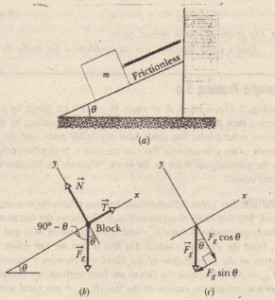# Sample Problem Force And Motion

Sample Problem Force And Motion

A  cord holds stationary a block of mass m = 15 kg, on a friction less plane that is inclined at angle 6 = 27°.
.a) What are the magnitudes of the force f On the block from the cord and the normal force N on the block from the plane?

SOLUTION: Those two forces and the gravitational force FR on the block are shown in the block’s free body diagram . Only these three forces . A Key Idea is that we can relate them to the block’s acceleration via Newton’s second  Law .We write asWith two unknown vector in , we cannot solve it for  either vector directly on a vector-capable calculator. So. we must rewrite it in terms of components. We use a coordinate system with its x axis parallel to the plane, as shown in then two forces (N and f) line up with the axes.

from which,(a) A block of mass m held stationaryby a cord. (b) A free-body diagram for the block. (c) The xand Y components.

(b) We now cut the cord. As the block then slides down the inclined plane, does it accelerate? If so, what is its acceleration?

SOLUTION: Cutting the cord removes force f from the block. Along the y axis, the normal force and component  are still in equilibrium. However, along the x axis, only force component  acts on the block; because it is directed down the plane (along the x axis), that component must cause the block to accelerate down the plane. Our Key Idea here is that we can relate  to the acceleration a that it produces with Newton’s second law written for x components. We getThe magnitude of this acceleration a is less than the magnitude of the free fall acceleration because only a component of the component that is directed down the plane is producing acceleration a.

CHECKPOINT 7: In the figure, horizontal force F is applied to a block on a ramp. (a) Is the component of F that is perpendicular to the ramp F cos 8 or F sin ? (b) Does the presence of F increase or decrease the magnitude of the normal force on the block from the ramp?